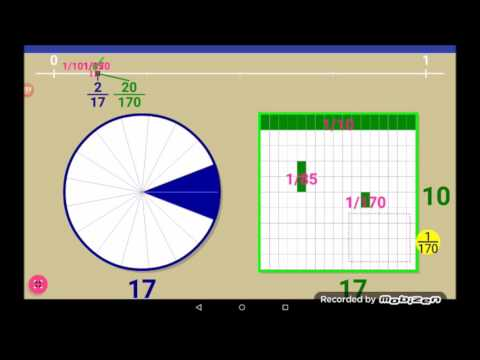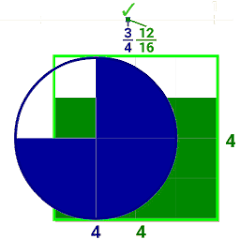# Proper Fractions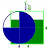1K+Everyone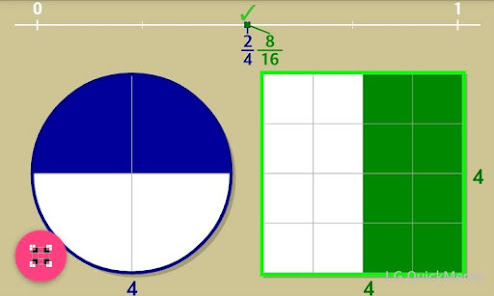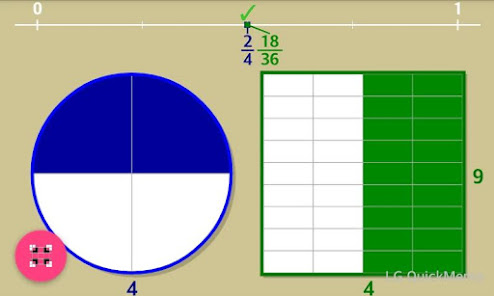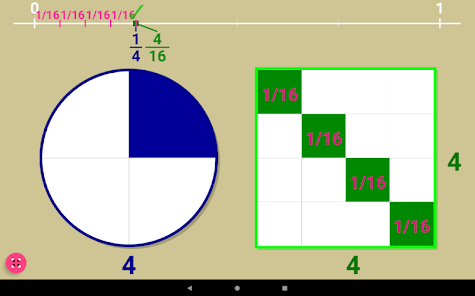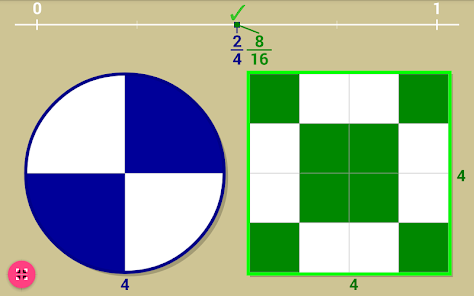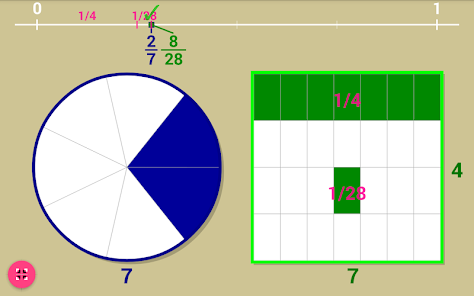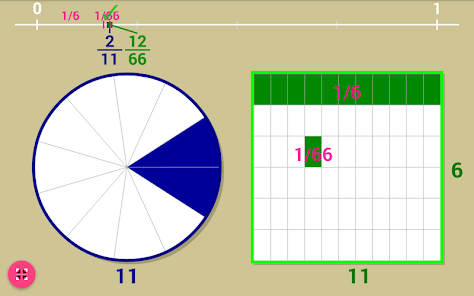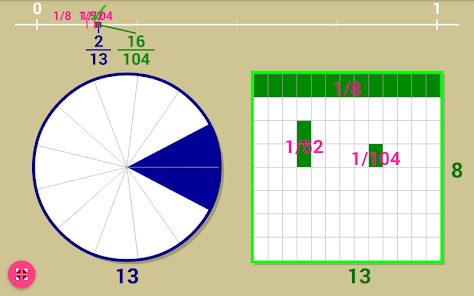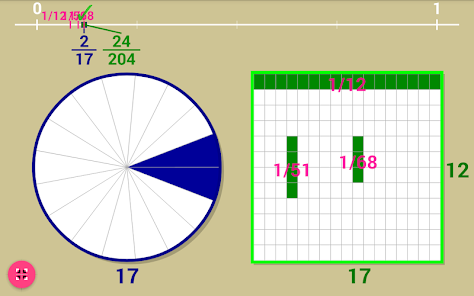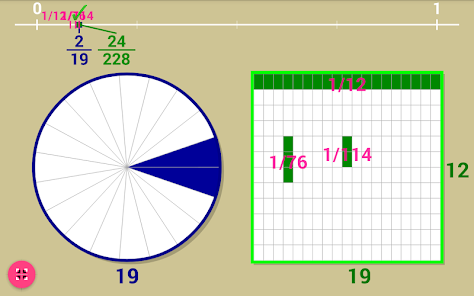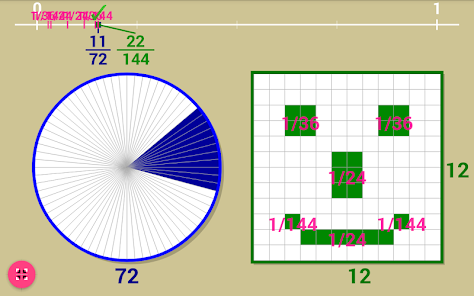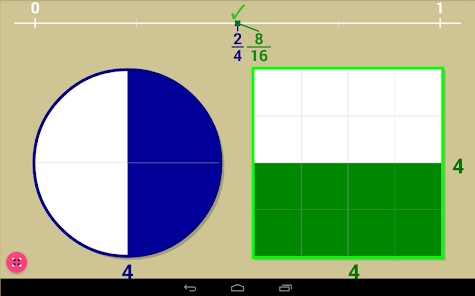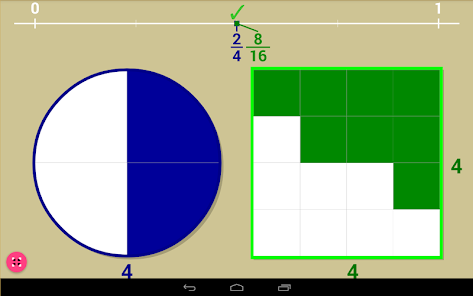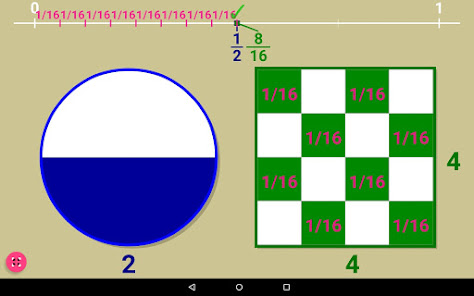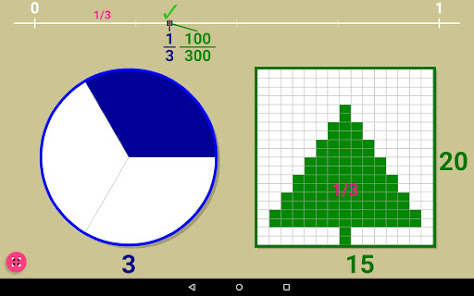Equivalent fractions (Using Proper Fractions)
Find corresponding Equivalent fractions:
Compare proper fractions in a circle with proper fractions in a square.
Increase or decrease the number of sections with 'pinch zoom'.
Open-ended explorations or guided options. (Select at: top bar)

(Or play with the Ahmes 2/n table of the RMP, like in the main video of the app)
(Or play with the table shown in the Akhmim Papyrus)
(Suggested by: Milo Gardner, Cryptanalyst)

PROPER FRACTIONS VIDEO PLAYLIST:
Suggested Proper Fractions Explorations:

NOTE: App Limits:
Square divisions limited to 31*31. (minimal unit fraction in square: 1/961)
Circle slice divisions limited to 1/101

Tool useful to solve problems from the following apps:
Adding Unit Fractions and A.U.F.+ (same developer)
Old Egyptian Fractions (mathcatsapps developer)

Registered at MathTools (MathForum)
http://mathforum.org/mathtools/tool/272979/
Courses:
Kindergarten, Math 1, 2, 3, 4, 5, 6, 7: Fractions, Equivalent fractions, Simplifying fractions, Adding fractions.

///////////////MATH NOTE//////////////////
Rhind Papyrus 2/n table:
2/3 = 1/2 + 1/6
2/5 = 1/3 + 1/15
2/7 = 1/4 + 1/28
2/9 = 1/6 + 1/18
2/11 = 1/6 + 1/66
2/13 = 1/8 + 1/52 + 1/104
2/15 = 1/10 + 1/30
2/17 = 1/12 + 1/51 + 1/68
2/19 = 1/12 + 1/76 + 1/114
2/21= 1/14 + 1/42
2/23 = 1/12 + 1/276
2/25 = 1/15 + 1/75
2/27 = 1/18 + 1/54
2/29 = 1/24 + 1/58 + 1/174 + 1/232
2/31 = 1/20 + 1/124 + 1/155
2/33 = 1/22 + 1/66
2/35 = 1/30 + 1/42
2/37 = 1/24 + 1/111 + 1/296
2/39 = 1/26 + 1/78
2/41 = 1/24 + 1/246 + 1/328
2/43 = 1/42 + 1/86 + 1/129 + 1/301
2/45 = 1/30 + 1/90
2/47 = 1/30 + 1/141 + 1/470
2/49 = 1/28 + 1/196
2/51 = 1/34 + 1/102
2/53 = 1/30 + 1/318 + 1/795
2/55 = 1/30 + 1/330
2/57 = 1/38 + 1/114
2/59 = 1/36 + 1/236 + 1/531
2/61 = 1/40 + 1/244 + 1/488 + 1/610
2/63 = 1/42 + 1/126
2/65 = 1/39 + 1/195
2/67 = 1/40 + 1/335 + 1/536
2/69 = 1/46 + 1/138
2/71 = 1/40 + 1/568 + 1/710
2/73 = 1/60 + 1/219 + 1/292 + 1/365
2/75 = 1/50 + 1/150
2/77 = 1/44 + 1/308
2/79 = 1/60 + 1/237 + 1/316 + 1/790
2/81 = 1/54 + 1/162
2/83 = 1/60 + 1/332 + 1/415 + 1/498
2/85 = 1/51 + 1/255
2/87 = 1/58 + 1/174
2/89 = 1/60 + 1/356 + 1/534 + 1/890
2/91 = 1/70 + 1/130
2/93 = 1/62 + 1/186
2/95 = 1/60 + 1/380 + 1/570 (not available in 'proper fractions' app)
2/97 = 1/56 + 1/679 + 1/776 (not available in 'proper fractions' app)
2/99 = 1/66 + 1/198 (not available in 'proper fractions app')
2/101 = 1/101 + 1/202 + 1/303 + 1/606 (not available in 'proper fractions' app)
Updated on
Sep 14, 2022

## Data safety

Safety starts with understanding how developers collect and share your data. Data privacy and security practices may vary based on your use, region, and age. The developer provided this information and may update it over time.No data shared with third parties